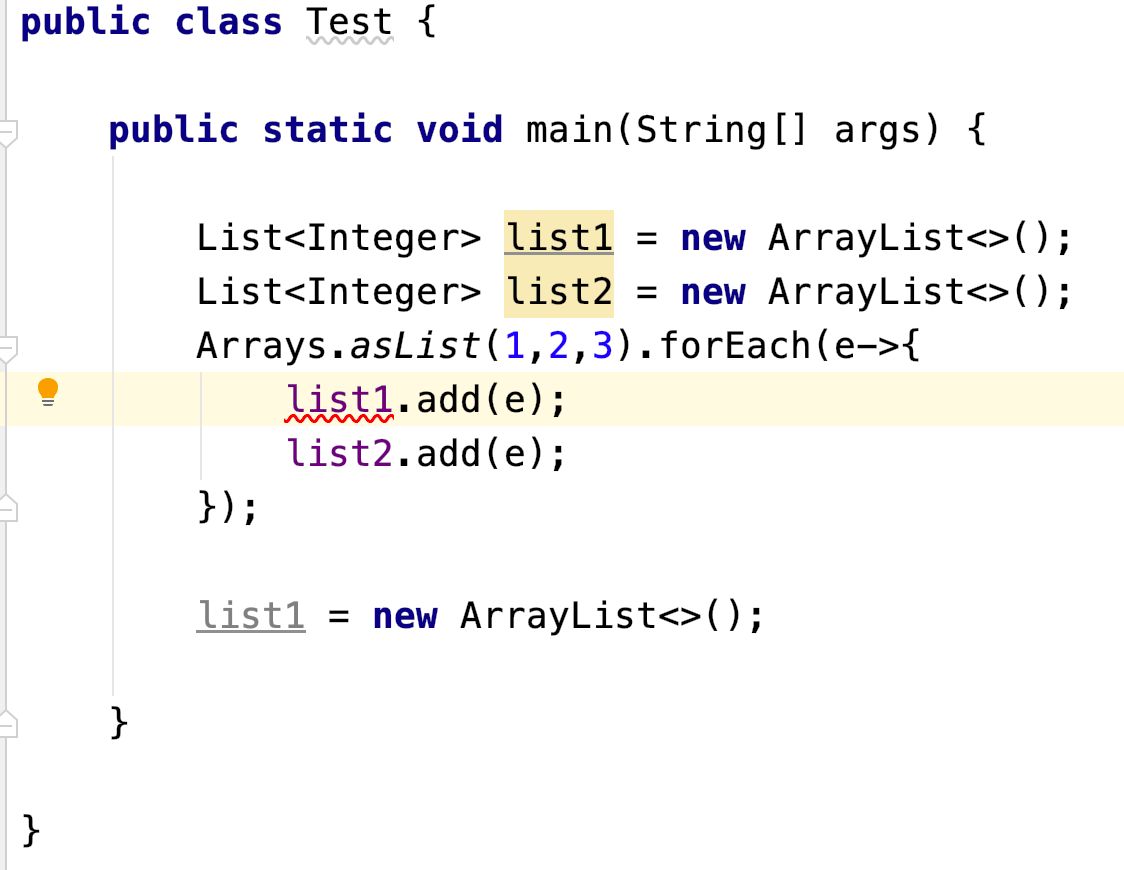# forEach中提示Copy xxx to effectively final temp variable原因

``````public class Test {

public static void main(String[] args) {

List<Integer> list1 = new ArrayList<>();
List<Integer> list2 = new ArrayList<>();
Arrays.asList(1,2,3).forEach(e->{
});

list1 = new ArrayList<>();

}

}
````````````public class Test {

public static void main(String[] args) {

List<Integer> list1 = new ArrayList<>();
final List<Integer> list2 = new ArrayList<>();
Arrays.asList(1,2,3).forEach(e->{
});

list1 = new ArrayList<>();

}

}
``````

``````public class Test {

public static void main(String[] args) {

final List<Integer> list1 = new ArrayList<>();
final List<Integer> list2 = new ArrayList<>();
Arrays.asList(1,2,3).forEach(e->{
});

list1 = new ArrayList<>();

}

}
``````